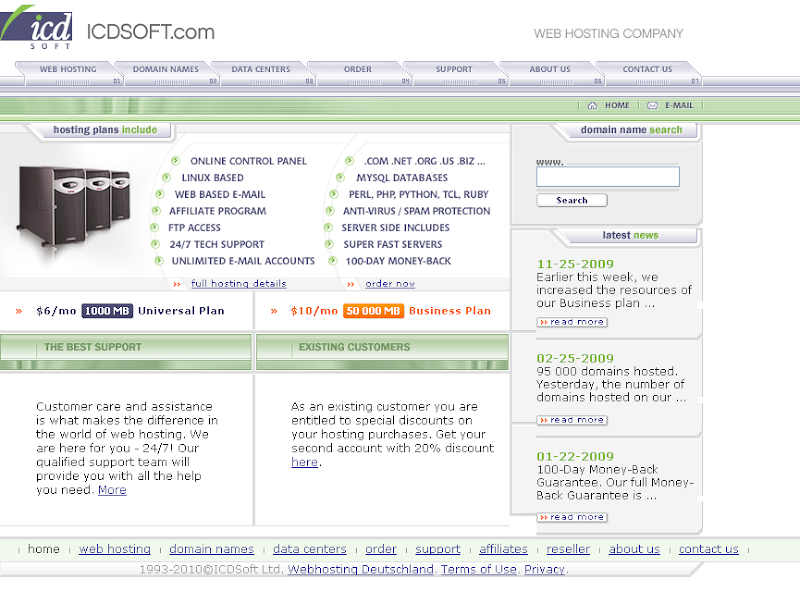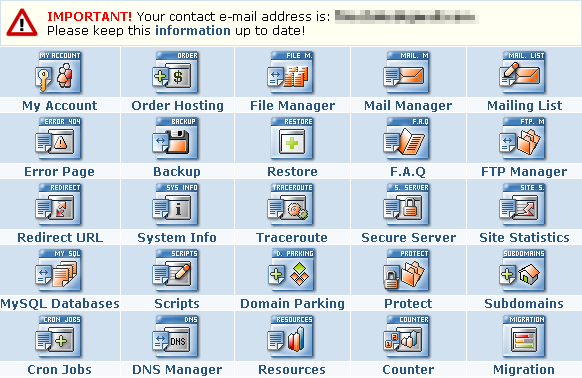換了虛擬主機商ping的結果讓我頗滿意，之前ping Bluehost、Lunarpages、HostGator都要250ms左右。

Reply from 203.194.200.22: bytes=32 time=71ms TTL=53
Reply from 203.194.200.22: bytes=32 time=71ms TTL=53
Reply from 203.194.200.22: bytes=32 time=71ms TTL=53
Reply from 203.194.200.22: bytes=32 time=70ms TTL=53
Reply from 203.194.200.22: bytes=32 time=70ms TTL=53
Reply from 203.194.200.22: bytes=32 time=71ms TTL=53
Reply from 203.194.200.22: bytes=32 time=76ms TTL=53
Reply from 203.194.200.22: bytes=32 time=70ms TTL=53
Reply from 203.194.200.22: bytes=32 time=69ms TTL=53
Reply from 203.194.200.22: bytes=32 time=70ms TTL=53
Reply from 203.194.200.22: bytes=32 time=71ms TTL=53Tags: , , ,